# animatplot¶

version: 0.2.2 Github

animatplot is a library for producing interactive animated plots in python built on top of matplotlib.

Contents

## Installation¶

Using pip:

pip install animatplot


Warning

If matplotlib was installed with anaconda, please upgrade matplotlib to >= 2.2 with anaconda before installing animatplot with pip. Otherwise, pip may butcher your environment(s).

If you are using jupyter lab, then install jupyter-matplotlib.

## Tutorial¶

### Getting Started¶

Animatplot is built on the concept of blocks. We’ll start by animating a Line block.

First we need some imports.

Note

Interactivity is not available in the static docs. Run the code locally to get interactivity.

#### Basic Animation¶

In :

%matplotlib notebook
import numpy as np
import matplotlib.pyplot as plt
import animatplot as amp


We will animate the function:

$$y = \sin(2\pi(x+t))$$ over the range $$x=[0,1]$$, and $$t=[0,1]$$

Let’s generate the data:

In :

x = np.linspace(0, 1, 50)
t = np.linspace(0, 1, 20)

X, T = np.meshgrid(x, t)
Y = np.sin(2*np.pi*(X+T))


In order to tell animatplot how to animate the data, we must pass it into a block. By default, the Line block will consider each of the rows in a 2D array to be a line at a different point in time.

We then pass a list of all our blocks into an Animation, and show the animation.

In :

block = amp.blocks.Line(X, Y)
anim = amp.Animation([block])

anim.save_gif('images/line1') # save animation for docs
plt.show()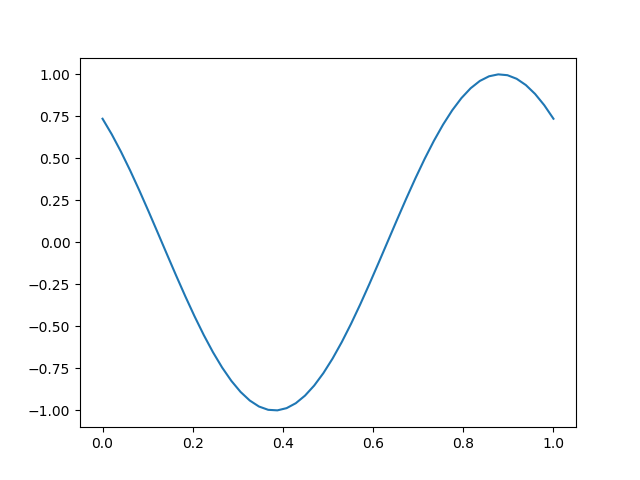We’ll use the same data to make a new animation with interactive controls.

In :

block = amp.blocks.Line(X, Y)
anim = amp.Animation([block])

anim.controls() # creates a timeline_slider and a play/pause toggle
anim.save_gif('images/line2') # save animation for docs
plt.show()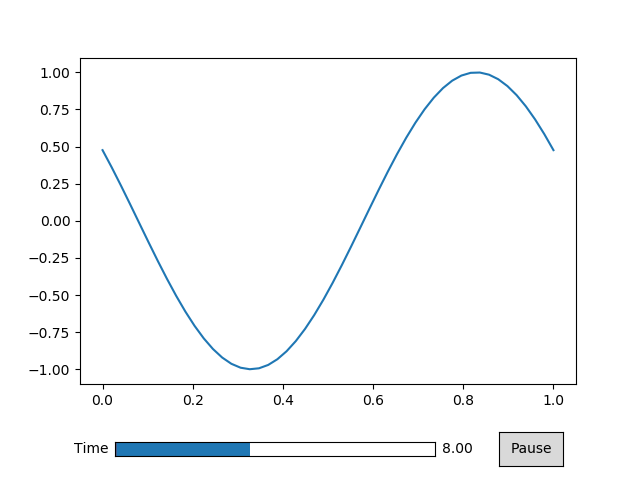#### Displaying the Time¶

The above animation didn’t display the time properly because we didn’t tell animatplot what the values of time are. Instead it displayed the frame number. We can simply pass our values of time into our call to Animation.

In :

block = amp.blocks.Line(X, Y)
anim = amp.Animation([block], t) # pass in the time values

anim.controls()
anim.save_gif('images/line3') # save animation for docs
plt.show()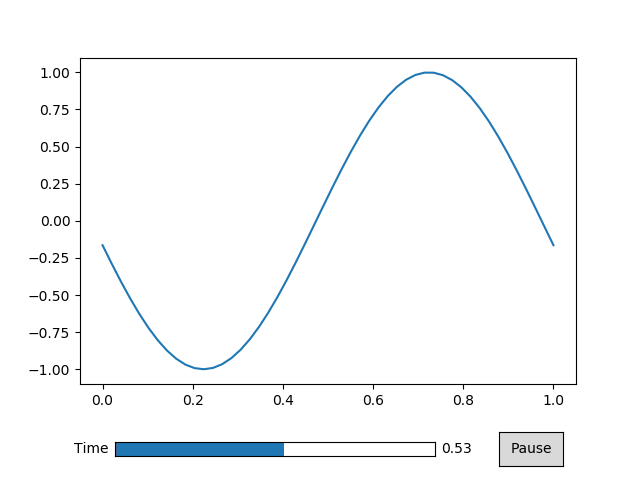#### Controlling Time¶

Simply passing in the values of time into the call to Animation doesn’t give us much control. Instead we use a Timeline.

In :

timeline = amp.Timeline(t, units='s', fps=20)


The units argument will set text to be displayed next to the time number.

The fps argument gives you control over how fast the animation will play.

In :

block = amp.blocks.Line(X, Y)
anim = amp.Animation([block], timeline) # pass in the timeline instead

anim.controls()
anim.save_gif('images/line4') # save animation for docs
plt.show()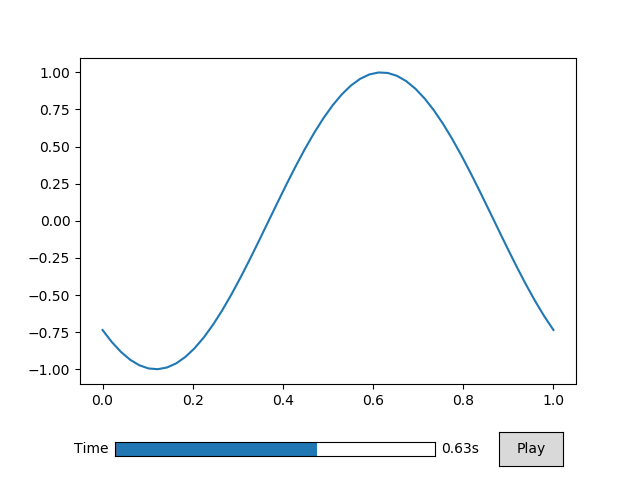#### Built on Matplotlib¶

Since animatplot is build on matplotlib, we can use all of our matplotlib tools.

In :

block = amp.blocks.Line(X, Y, marker='.', linestyle='-', color='r')
anim = amp.Animation([block], timeline)

# standard matplotlib stuff
plt.title('Sine Wave')
plt.xlabel('x')
plt.ylabel('y')

anim.controls()
anim.save_gif('images/line5') # save animation for docs
plt.show()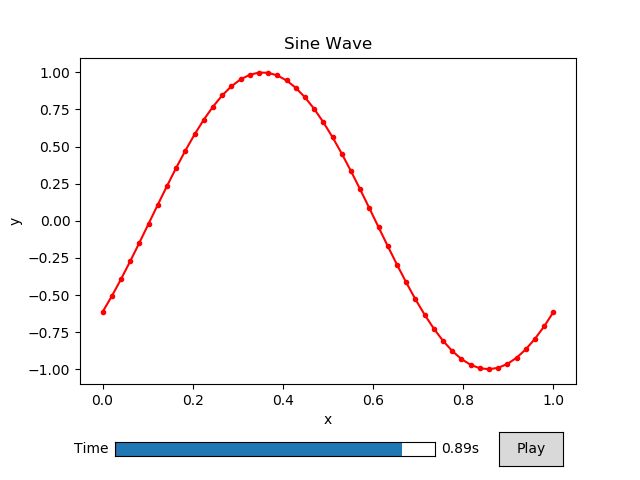### Using multiple blocks¶

Here we are going to use 2 different blocks in our animation.

First we need some imports:

In :

%matplotlib notebook
import numpy as np
import matplotlib.pyplot as plt
import animatplot as amp


We are going to plot a pcolormesh and a line on 2 different axes.

Let’s use: $$z = \sin(x^2+y^2-t)$$ for the pcolormesh, and a cross-section of $$y=0$$: $$z = \sin(x^2-t)$$ for the line.

First, we generate the data.

In :

x = np.linspace(-2, 2, 41)
y = np.linspace(-2, 2, 41)
t = np.linspace(0, 2*np.pi, 30)

X, Y, T = np.meshgrid(x, y, t)

pcolormesh_data = np.sin(X*X+Y*Y-T)
line_data       = pcolormesh_data[20,:,:] # the slice where y=0


We need to be careful here. Our time axis is the last axis of our data, but animatplot assumes it is the first axis by default. Fortunately, we can use the t_axis argument.

We use the axis argument to attached the data to a specific subplot.

In :

# standard matplotlib stuff
# create the different plotting axes
fig, (ax1, ax2) = plt.subplots(1, 2)

for ax in [ax1, ax2]:
ax.set_aspect('equal')
ax.set_xlabel('x')

ax1.set_ylabel('z')
ax1.set_ylim([-1.1,1.1])

fig.suptitle('Multiple blocks')
ax1.set_title('Cross Section: $y=0$')
ax2.set_title(r'$z=\sin(x^2+y^2-t)$')

# animatplot stuff
# now we make our blocks
line_block       = amp.blocks.Line(X[0,:,:], line_data,
axis=ax1, t_axis=1)
pcolormesh_block = amp.blocks.Pcolormesh(X[:,:,0], Y[:,:,0], pcolormesh_data,
axis=ax2, t_axis=2, vmin=-1, vmax=1)
timeline = amp.Timeline(t, fps=10)

# now to contruct the animation
anim = amp.Animation([pcolormesh_block, line_block], timeline)
anim.controls()

anim.save_gif('images/multiblock')
plt.show()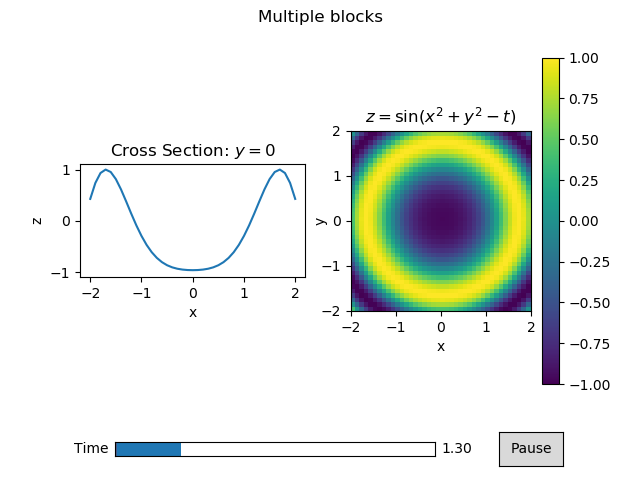There is a lot going on here so lets break it down.

Firstly, the standard matplotlib stuff is creating, and labeling all of our axes for our subplot. This is exactly how one might do a static, non-animated plot.

When we make the Line block, we pass in the data for our lines as 2D arrays (X[0,:,:] and line_data). We attached that line to the first axis axis=ax1. We also specifify that the time axis is the last axis of the data t_axis=1.

When we make the Pcolormesh block, we pass in the x, y data as 2D arrays (X[:,:,0] and Y[:,:,0]), and the z data as a 3D array. We attached the pcolormesh to the second axis axis=ax2. We also specifify that the time axis is the last axis of the data t_axis=2.

Additional, we told the Pcolormesh blocks what the minimum and maximum values will be (vmin=-1 and vmax=1), so that the colorscale will be proper. The keywords vmin, and vmax get passed to the underlaying called to matplotlib’s pcolormesh.

plt.colorbar does not recognize the Pcolormesh block as a mappable, so we pass in a mappable from the block to get the colorbar to work. In the future, animatplot may have a wrapper around this.

The rest simply brings all of the blocks, and the timeline together into an animation.### Custimizing the Controls¶

Here we’ll like how to manipulate the timeline_slider and the toggle button.

The interactive controls can be make using the controls() method of the animation class, as in the getting started tutorial, but this method is a wrapper around the toggle and timeline_slider methods.

First, we need from imports and data to animate.

In :

%matplotlib notebook
import numpy as np
import matplotlib.pyplot as plt
import animatplot as amp

In :

x = np.linspace(0, 1, 50)
t = np.linspace(0, 1, 20)

X, T = np.meshgrid(x, t)
Y = np.sin(2*np.pi*(X+T))

Animation.toggle(axis=None)[source]

Creates a play/pause button to start/stop the animation

Parameters: axis (optional) – A matplotlib axis to attach the button to.
Animation.timeline_slider(axis=None, valfmt=’%1.2f’, color=None)[source]

Creates a timeline slider.

Parameters: axis (optional) – A matplotlib axis to attach the slider to valfmt (str, optional) – a format specifier used to print the time Defaults to ‘%1.2f’ color – The color of the slider.
Animation.controls(timeline_slider_args={}, toggle_args={})[source]

Creates interactive controls for the animation

Creates both a play/pause button, and a time slider at once

Parameters: timeline_slider_args (Dict, optional) – A dictionary of arguments to be passed to timeline_slider() toggle_args (Dict, optional) – A dictionary of argyments to be passed to toggle()

Now to make the animation

By specifying the axis parameter, we can change the position of either the toggle or the timeline_slider.

We use color to change the color of the slider, and valfmt to change how the time is displayed.

Let’s create our block, then create the controls at the top of the animation.

In :

block = amp.blocks.Line(X, Y)

plt.subplots_adjust(top=0.8) # squish the plot to make space for the controls
slider_axis = plt.axes([.18, .89, .5, .03]) # the rect of the axis
button_axis = plt.axes([.78, .87, .1, .07]) # x, y, width, height

anim = amp.Animation([block])

anim.toggle(button_axis)
anim.timeline_slider(slider_axis, color='red', valfmt='%1.0f')
# equivalent to:
# anim.controls({'axis':slider_axis, 'color':'red', 'valfmt': '%1.0f'},
#               {'axis':button_axis})

anim.save_gif('images/controls')
plt.show()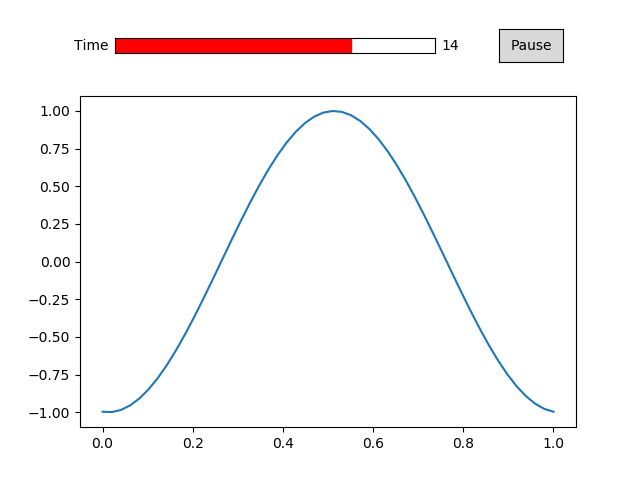### Using Jupyter¶

In order to display interactive animations in jupyter notebook or lab, use one of the following line magics:

%matplotlib notebook  # notebook only
%matplotlib ipympl  # notebook or lab
%matplotlib widget  # notebook or lab (equivalent to ipympl)


## API¶

Animatplot is build on top of three main classes:

• Animation
• Block
• Timeline

A Timeline holds the information and logic to actually control the timing of all animations.

A Block represent any “thing” that is to be animated.

An Animation is a composition of a list of blocks and a timeline. This class builds the final animation.

### Animation¶

 Animation(blocks[, timeline, fig]) The foundation of all animations.

### Timeline¶

 Timeline(t[, units, fps, log]) An object to contain and control all of the time

### blocks¶

Blocks handle the animation of different types of data. The following blocks are available in animatplot.blocks.

 Block([axis, t_axis]) A base class for blocks Line(x, y[, axis, t_axis]) Animates lines Quiver(X, Y, U, V[, axis, t_axis]) A block for animated quiver plots Pcolormesh(*args[, axis, t_axis]) Animates a pcolormesh Imshow(images[, axis, t_axis]) Animates a series of images Nuke(func, axis, length[, fargs]) For when the other blocks just won’t do

## Developer Setup¶

### Requirements¶

The following are required to build the docs.

sphinx>=1.5.1
ipykernel
nbsphinx
matplotlib>=2.2
numpy


### Install¶

Clone and install the repository:

git clone https://github.com/t-makaro/animatplot.git
cd animatplot
pip install -e .


### Testing¶

From the root animatplot directory simply run:

pytest


Warning

Tests are currently very limited. Please run examples to ensure everything works.

### Linting¶

This project currently uses pycodestyle for linting.

## Changes to animatplot¶

### 0.2.2¶

• Fix .animations and .blocks subpackages not being distributed properly.

### 0.2.0¶

• Complete and total overhaul of animatplot using with the idea of blocks as a foundation
• Chuck all previous attempts to support python 2 in the dumpster

### 0.1.0.dev3¶

This is the original release.